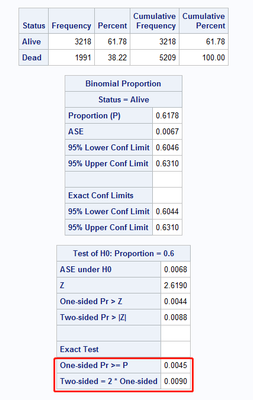## Proc Freq Exact binomial Test p-value

when i do the Exact Binomial Test with PROC FREQ (H0: P<=P0, H1: P>P0), i can obtain the p-value as:

One-sided Pr <= P

or

One-sided Pr >= P

depending on the Z statistics value.

having doing some checks with other softwares, i need to use One-sided Pr >= P but One-sided Pr >= P  is not equal to 1 - One-sided Pr <= P because the part of the "=" is included in both p-values.

1) So is there a way to force SAS to always present the p-value in the same way (i.e. always One-sided Pr >= P for example)?

2) if you are interested by "One-sided Pr >= P", how do you manage to have the correct p-value because when i have the p-value "One-sided Pr <= P", i cannot simply do "1-One-sided Pr <= P".

Thanks.

7 REPLIES 7

## Re: Proc Freq Exact binomial Test p-value

Hello @AudreyM

You could do a trick and actually perform a non-inferiority test with margin zero. PROC FREQ doesn't allow margin zero, so use an extremely small positive margin, e.g., 1E-99.

Example:

```* Create sample data for demonstration */

data have;
do i=1 to 20;
x=(i>9);
output;
end;
run;

/* Compute exact p-value for H0: P<=0.6 vs. H1: P>0.6 */

proc freq data=have;
tables x / binomial(level='1' p=0.6 noninf margin=1e-99);
exact binomial;
run;```

Result:

```                Noninferiority Analysis

H0: P - p0 <= -Margin    Ha: P - p0 > -Margin

p0 = 0.6   Margin = 1E-99

Proportion         ASE                           Exact
(P)    (Sample)          Z    Pr > Z    Pr >= P

0.5500      0.1112    -0.4495    0.6735     0.7553```

Just to confirm the result:

```data _null_;
p=sdf('binom',10,0.6,20);
put p= best16.;
run;```
`p=0.75533720331639`

To extend the check beyond the fourth decimal, add

`ods output binomialnoninf=bt;`

before the PROC FREQ step. Then check variable XPVALUE in dataset BT:

```proc print data=bt;
format xpvalue best16.;
run;```

Again:

```         XPValue

0.75533720331639```

## Re: Proc Freq Exact binomial Test p-value

If I understand your question, you are running code something like this:

``````data Have;
call streaminit(1);
do i = 1 to 100;
x = rand("Bern", 0.6);
output;
end;
run;

proc freq data=Have;
tables x / binomial(level='1' p=0.6);
exact binomial;
run;
``````

Perhaps you can refer to the output of this program (or a modified version of it) to explain what problem you are trying to resolve.

The documentation for PROC FREQ includes a section on "Binomial Test" and "Equality Test".

I don't understand your question about "forcing SAS to always present the p-values in the same way." The definition of p-values are standard. For a discrete distribution (such as the binomial distribution), they include the probability of the bar that includes the null value of the hypothesis.

If you want some probabilities to sum to 1, then make sure they are disjoint. You can subtract the probability density for the null value to get
P(X < value) + P(X = value) + P(X > value) = 1.Ksharp
Super User

## Re: Proc Freq Exact binomial Test p-value

I think @FreelanceReinh nailed it .

If you want test your hypothesis (H0: P<=P0, H1: P>P0) you need perform a non-inferiority test which is one-side test .

``````/*
Can you post your code and output ?
And I don't understand your question.
I think your hypothesis should be
(H0: P=P0, H1: P>P0)     <-- for one side
(H0: P=P0, H1: P not equal P0)  <-- for two side

Therefore, Two-sided = 2 * One-sided

"One-sided Pr <= P"  should be equal  "One-sided Pr >= P" ,since distribution of P is symmetry .
*/
proc freq data=sashelp.heart;
table status/binomial(level='Alive' p=0.6);
exact binomial;
run;``````## Re: Proc Freq Exact binomial Test p-value

A superiority test works as well:

`... binomial(level='1' p=0.6 margin=1e-99 sup);`

For the other direction you could use an equivalence test

`... binomial(level='1' p=0.6 margin=1e-99 equiv);`

as this would always yield two p-values:

```                  Two One-Sided Tests (TOST)

Test                   Z        P-Value         Exact P-Value

Lower Margin     -0.4495    Pr > Z   0.6735    Pr >= P   0.7553
Upper Margin     -0.4495    Pr < Z   0.3265    Pr <= P   0.4044```

(using the sample data from my previous post).

Equality of these two p-values would occur for the symmetric binomial distribution, i.e., p=0.5, if, in addition, the observed frequency is the expected value np, which requires an even n. For example, n=20, p=0.5, 10 "successes" observed:

```  Exact P-Value

Pr >= P   0.5881
Pr <= P   0.5881```Ksharp
Super User

## Re: Proc Freq Exact binomial Test p-value

"A superiority test works as well:"
Yes. since margin is so small (near zero), and would expect they have similar result.

## Re: Proc Freq Exact binomial Test p-value

@FreelanceReinh wrote:

Equality of these two p-values would occur for the symmetric binomial distribution, i.e., p=0.5, if, in addition, the observed frequency is the expected value np, which requires an even n.

These conditions are sufficient, but not necessary for the equality. There are many other examples, e.g., n=5, 1 success observed, p such that (1-p)**5=1-(1-p)**5-5*p*(1-p)**4 -- this equation has the unique solution p=0.216105706313... in [0, 1]. Both one-sided p-values are then 0.7040028597...Ksharp
Super User

## Re: Proc Freq Exact binomial Test p-valueDiscussion stats
• 7 replies
• 335 views
• 2 likes
• 4 in conversation